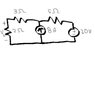# Superposition theorem

• vs55

## Homework Statement

Use the superposition theorem to find Vo in the circuit

## The Attempt at a Solution

i made 8A source 0 so the first equation using KVL becomes:
10i1 = -20
i1 = -2
then making the 20V source 0, the next KVL equation becomes:
i think it's 5i2-8 = 0
i2 = 8/5

i think I'm messing up the second equation with the 20V source as 0...

#### Attachments

•Untitled.jpg
10.2 KB · Views: 435
Last edited: